# Historical Categories

## AverageNumberPerRelativeHour

This statistical category returns the number of events of a particular type that occurred during an average hour. Here is the formula: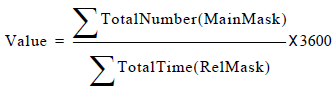A relative mask specification is mandatory for this category. The subject applies to both MainMask and RelMask. Filters, however, can only be applied to the MainMask.

Here is an example of a stat-type definition using the AverageNumberPerRelativeHour statistical category:
[Average_Calls_Per_Hour]
Subject = AgentStatus
Category = AverageNumberPerRelativeHour
Objects = GroupAgents, GroupPlaces, Agent, Place

A statistic based on this stat-type definition collectively averages all types of calls that agents receive over the time they are both monitored (~NotMonitored) and not logged out (~LoggedOut) or, in other words, logged in.

Important
Filter for this category should not be used with Subject=AgentStatus, Subject=DNStatus, or Subject=PlaceStatus. However, you can use a business attribute. For example, the MediaType=chat business attribute can be added to a stat type definition to calculate only chat interactions.

## AverageOfCurrentNumber

The value represents the average of current-number measurements over a specified time interval. Unlike the behavior in releases prior to 7.0, Stat Server take current-number measurements every 2 seconds; for example, Stat Server now only notes the time whenever the current number changes—for example, when one inbound interaction enters or exits the contact center. Also, different from prior releases is Stat Server’s use of the first-observed current number in its average calculation. Prior to release 7.0, the first value was not used in the formula. The new formula: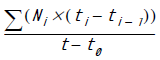considers this value. When the denominator is 0, Stat Server returns 0.

Figure below illustrates how an AverageOfCurrentNumber statistic might be depicted in a graph. In this figure, N denotes the number of interactions currently underway. When a new current interaction, N+1, enters the contact center, Stat Server timestamps it, represented by the corresponding time value along the x-axis. The variable i serves as a change index in the number of interactions entering or leaving the contact center. Point (Ni,ti), then, denotes the number of interactions currently underway at a specific time during the ith change in the number of current observations.

## AverageOfCurrentTime

The value is equal to the average of current-time measurements and is calculated similarly to how AverageOfCurrentNumber (see previous subsection) is measured. Unlike the behavior in releases prior to 7.0, Stat Server now uses the first-observed current time in its average calculation.

## AverageTime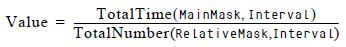When the denominator is 0, the returned value is 0. The value is the quotient of the TotalTime aggregated value for the main mask and the TotalNumber aggregated value for the relative mask.

## ElapsedTimePercentage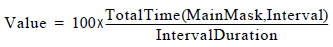This category returns, as a percentage, the quotient of the TotalTime aggregated value and the entire duration of the interval from which the statistic is calculated. Note that this percentage can exceed 100 if some of the actions in the main mask occur simultaneously on the object for which a statistic for this category is calculated. It can also exceed 100 when actions that start before the beginning of the interval from which the statistic is calculated end during that interval.

## MaxNumber

The MaxNumber statistical category returns an aggregated value that represents the maximum number of durable actions or statuses that occur simultaneously during an interval.

## MaxTime

The MaxTime statistical category returns an aggregated value that represents the maximum duration among all durations of durable and retrospective actions or of statuses listed in the mask that, during the interval from which the statistic is calculated:

• Ended (for durable actions).
• Occurred (for retrospective actions).
• Either started or are in progress (for statuses).

Momentary actions listed in the mask are ignored, because they do not have a duration. If a statistic is requested for statuses, Stat Server uses the status duration within the statistical interval for calculation; otherwise, Stat Server uses the entire action duration.

## MinNumber

The MinNumber statistical category returns an aggregated value that represents the minimum number of durable actions or statuses that occur simultaneously during an interval.

## MinTime

The MinTime statistical category returns an aggregated value that represents the minimum duration among all durations of durable and retrospective actions or of statuses listed in the mask that, during the interval from which the statistic is calculated:

• Ended (for durable actions).
• Occurred (for retrospective actions).
• Either started or are in progress (for statuses).

Momentary actions listed in the mask are ignored, because they do not have a duration. If a statistic is requested for statuses, Stat Server uses the status duration within the statistical interval for calculation; otherwise, Stat Server uses the entire action duration.

## RelativeNumberPercentage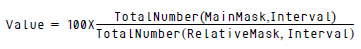When the denominator is 0, the returned value is 0.

A relative mask is required for this category. It returns, as a percentage, the quotient of the TotalNumber aggregated value for the main mask and the TotalNumber aggregated value for the relative mask. Note that if the main mask contains actions absent from the relative mask, the percentage can exceed 100.

In addition, you can specify a time range for a statistic of this category. When specified, Stat Server applies the time range to the actions/statuses that define the main mask for this statistic. So, in this case, the numerator for this category is calculated as:

Refer to Aggregated Values using TimeRanges for additional information about historical statistical categories using time ranges.

## RelativeTimePercentage

A relative mask is required for this category. It returns, as a percentage, the quotient of the TotalTime aggregated value for the main mask and the TotalTime aggregated value for the relative mask. Note that if the main mask contains actions absent from the relative mask, the percentage can exceed 100.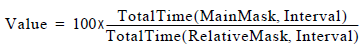When the denominator is 0, the returned value is 0.

## TotalNumber

For statistics based on stat type definitions where Subject=DNAction, the TotalNumber statistical category returns an aggregated value that represents the total number of actions listed in the mask that ended (for durable actions) or occurred (for instantaneous actions) during the interval from which the statistic is calculated. For statistics based on stat type definitions where Subject= DNStatus (and, respectively, AgentStatus and PlaceStatus), this is the total number of statuses listed in the mask that either started or are in progress during the interval from which the statistic is calculated.

The TotalAdjustedNumber statistical category sums the total number of occurrences of actions or statuses listed in the main mask that ended during the interval from which the statistic is calculated.

Important
The TotalAdjustedNumber category differs from TotalNumber only if reset-based notification is used for the statistic. For all other notification modes, TotalAdjustedNumber values are identical to TotalNumber values.

If a statistic is requested with the DN action specified, TotalAdjustedTime represents the sum of all durations of durable and retrospective actions listed in the mask that, during the interval from which the statistic is calculated:

• Either ended or are in progress (for durable actions)
• Occurred (for retrospective actions)

Momentary actions listed in the mask are ignored, because they do not have a duration. Only the duration time that is within the interval for durable actions and whole duration for retrospective actions are used in this calculation.

For status-based statistics, TotalAdjustedTime is the sum of all durations of statuses listed in the mask that ended during the interval from which the statistic is calculated.

Stat Server uses the overall status duration in this calculation. A statistic of this category must be requested with the reset-based notification; that is, a statistic is reset to 0 when a new interval starts.

Important
• The TotalAdjustedTime category differs from TotalTime only if reset-based notification is used for the statistic. For all other notification modes, TotalAdjustedTime values are identical to TotalTime values.
• For a DNAction or Action subject, TotalAdjustedTime reports finished and unfinished actions for the current interval.
• For the DNStatus and AgentStatus subjects, however, TotalAdjustedTime causes Stat Server to report the entire time a DN or agent status occurs only if it ends within the time interval.
• TotalAdjustedTime calculation for retrospective actions was corrected in 8.5 Release.

## TotalContinuousNumber

At any moment in time, the TotalContinuousNumber is the sum of the current (C) and historical (H) components:

V = C + H,

where

• V is the value of the statistic, sent to the client;
• C coincides with the value of the CurrentNumber statistic with the same MainMask and Subject;
• H is invisible internal counter.

Current component C is equal to 1, if current object status belongs to MainMask, and zero (0), otherwise.

Historical component H increases by 1 every time, when status is changed from value in MainMask to value not in MainMask.

In more details:

• When object status changes from S1 (not in MainMask) to S2 (not in MainMask), it does not affect the C, H;
• When object status changes from S1 (not in MainMask) to S2 (in MainMask), C is 1 and V is V+1;
• When status changes from S1 (in MainMask) to S2 (in MainMask), it does not affect the C, H;
• When object status changes from S1 (in MainMask) to S2 (not in MainMask), C is zero (0), H is H+1, and V is unchanged;
• When interval ends H is zero (0), C is unchanged, and V is equal C.
Important
Filter for this category should not be used with Subject=AgentStatus, Subject=DNStatus, or Subject=PlaceStatus. However, you can use a business attribute. For example, the MediaType=chat business attribute can be added to a stat type definition to calculate only chat interactions.

## TotalNumberInTimeRange

TotalNumberInTimeRange returns a restricted aggregated value that represents the total number of all durable and retrospective actions, or of statuses listed in the mask that ended (for durable actions or for statuses) or occurred (for retrospective actions) during the interval from which the statistic is calculated, and whose duration is within the specified time range.

## TotalNumberInTimeRangePercentage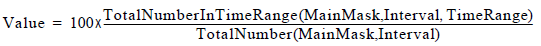When the denominator is 0, the returned value is 0.

A time range is required for this category. It returns, as a percentage, the ratio of the restricted TotalNumberInTimeRange aggregated value and the TotalNumber aggregated value—that is, the percentage of times the actions in the main mask had a duration within the specified time range over the total number of times actions from the main mask occurred or ended during the specified interval.

## TotalNumberPerSecond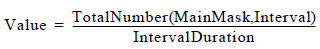This category returns the ratio of the TotalNumber aggregated value to the entire duration of the interval, from which the statistic is calculated.

## TotalTime

The TotalTime statistical category returns an aggregated value that represents the sum of all durations of durable and retrospective actions or of statuses listed in the mask that, during the interval from which the statistic is calculated:

• Ended (for durable actions).
• Occurred (for retrospective actions).
• Either started or are in progress (for statuses).

Momentary actions listed in the mask are ignored, because they do not have a duration. If a statistic is requested for statuses, Stat Server uses the status duration within the statistical interval for calculation; otherwise, Stat Server uses the entire action duration.